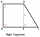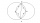# Expression of a variable from the formula + Pythagorean theorem - math problems

#### Number of problems found: 293

• Right triangleIt is given a right triangle angle alpha of 90 degrees beta angle of 55 degrees c = 10 cm use Pythagorean theorem to calculate sides a and b
• CalculateCalculate the length of a side of the equilateral triangle with an area of 50cm2.
• Circle - AGFind the coordinates of circle and its diameter if its equation is: ?
• On a lineOn a line p : 3 x - 4 y - 3 = 0, determine the point C equidistant from points A[4, 4] and B[7, 1].
• On lineOn line p: x = 4 + t, y = 3 + 2t, t is R, find point C, which has the same distance from points A [1,2] and B [-1,0].
• PilotHow high is the airplane's pilot to see 0.001 of Earth's surface?
• Calculate 7Calculate the height of the trapezoid ABCD, where coordinates of vertices are: A[2, 1], B[8, 5], C[5, 5] and D[2, 3]
• Five circlesOn the line segment CD = 6 there are 5 circles with radius one at regular intervals. Find the lengths of the lines AD, AF, AG, BD, and CE
• Spherical cap 4What is the surface area of a spherical cap, the base diameter 20 m, height 2.5 m? Calculate using formula.
• Height of pyramidThe pyramid ABCDV has edge lengths: AB = 4, AV = 7. What is its height?The ladder is 10 m long The ladder is 8 m high How many meters is the distant heel from the wall?
• Calculate 6Calculate the distance of a point A[0, 2] from a line passing through points B[9, 5] and C[1, -1].
• Above EarthTo what height must a boy be raised above the earth in order to see one-fifth of its surface.
• Diagonal of squareCalculate the side of a square when its diagonal is 10 cm.
• Integer sidesA right triangle with an integer length of two sides has one leg √11 long. How much is its longest side?
• Equilateral triangleCalculate the area of an equilateral triangle with circumference 72cm.How long is a ladder that touches on a wall 4 meters high and its lower part is 3 meters away from the wall?
• RT 11Calculate the area of right tirangle if its perimeter is p = 45 m and one cathethus is 20 m long.
• TetrahedronCalculate height and volume of a regular tetrahedron whose edge has a length 4 cm.
• Two circlesTwo circles with the same radius r = 1 are given. The center of the second circle lies on the circumference of the first. What is the area of a square inscribed in the intersection of given circles?

Do you have an interesting mathematical word problem that you can't solve it? Submit a math problem, and we can try to solve it.

We will send a solution to your e-mail address. Solved examples are also published here. Please enter the e-mail correctly and check whether you don't have a full mailbox.

Please do not submit problems from current active competitions such as Mathematical Olympiad, correspondence seminars etc...

Pythagorean theorem is the base for the right triangle calculator.